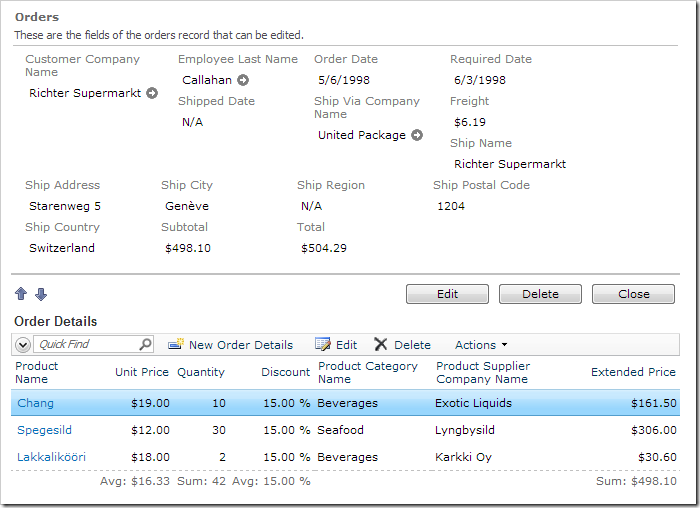# Blog: Posts from October, 2012

Labels
Archive
Blog
Posts from October, 2012
Tuesday, October 30, 2012PrintSubscribe
Order Form Sample–Part 25

The calculation will analyze Order ID and current Freight value:

If the order total is greater than \$100, then Freight will be \$19.95 flat.
Otherwise, Freight is \$3.95.

The Order Form page will allow overriding the Freight value.

In the Project Explorer, double-click on Orders / Fields / Freight (Decimal) field node.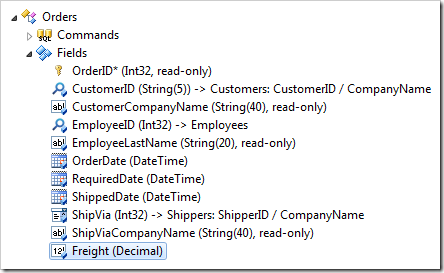Change the Context Fields:

 Property New Value Context Fields OrderDetails,Freight

Press OK to save.

If you implemented an SQL Business Rule to calculate Subtotal and Total, continue reading the next section. If you implemented the C# / Visual Basic Business rule, skip the next section. You may also use JavaScript – skip the next two sections.

In the Project Explorer, double-click on Orders / Business Rules / Calculate (Sql / Execute) node.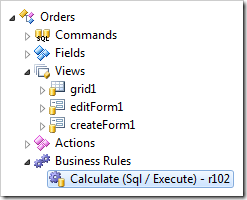Append the script:

 Property New Value Script ```select @Subtotal = sum(unitprice * quantity * (1 - discount)) from [Order Details] where OrderID = @OrderID set @Total = @Subtotal + @Freight if (@Freight is not null or @Freight = 0 or @Freight = 3.95 or @Freight = 19.95) begin if (@Total > 100) set @Freight = 19.95 else set @Freight = 3.95 end```

Press OK to save.

Right-click on Orders / Business Rules / Calculate (Code / Execute) node, and select Edit Rule in Visual Studio.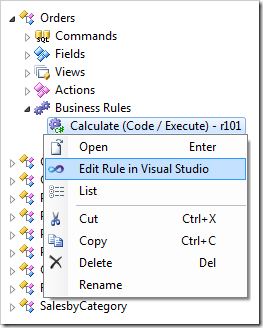Append the following code.

C#:

```using System;
using MyCompany.Data;

namespace MyCompany.Rules
{
{

[Rule("r101")]
public void r101Implementation(
int? orderID,
string customerID,
string customerCompanyName,
int? employeeID,
string employeeLastName,
DateTime? orderDate,
DateTime? requiredDate,
DateTime? shippedDate,
int? shipVia,
string shipViaCompanyName,
decimal? freight,
string shipName,
string shipCity,
string shipRegion,
string shipPostalCode,
string shipCountry,
decimal? subtotal)
{
object total = null;
using (SqlText calc = new SqlText(@"select sum(unitprice *
quantity * (1 - discount)) from
[Order Details] where OrderID= @OrderID"))
{
total = calc.ExecuteScalar();
}

if (DBNull.Value.Equals(total))
{
UpdateFieldValue("Subtotal", 0);
UpdateFieldValue("Total", 0);
}
else
{
UpdateFieldValue("Subtotal", Convert.ToDecimal(total));
UpdateFieldValue("Total", Convert.ToDecimal(total) + freight);
}

if (!freight.HasValue || freight.Value == 0 ||
freight.Value == 3.95m || freight.Value == 19.95m)
if (Convert.ToDecimal(total) > 100)
UpdateFieldValue("Freight", 19.95);
else
UpdateFieldValue("Freight", 3.95);
}
}
}```

Visual Basic:

```Imports MyCompany.Data
Imports System

Namespace MyCompany.Rules

<Rule("r101")> _
Public Sub r101Implementation( _
ByVal orderID As Nullable(Of Integer), _
ByVal customerID As String, _
ByVal customerCompanyName As String, _
ByVal employeeID As Nullable(Of Integer), _
ByVal employeeLastName As String, _
ByVal orderDate As Nullable(Of DateTime), _
ByVal requiredDate As Nullable(Of DateTime), _
ByVal shippedDate As Nullable(Of DateTime), _
ByVal shipVia As Nullable(Of Integer), _
ByVal shipViaCompanyName As String, _
ByVal freight As Nullable(Of Decimal), _
ByVal shipName As String, _
ByVal shipCity As String, _
ByVal shipRegion As String, _
ByVal shipPostalCode As String, _
ByVal shipCountry As String, _
ByVal subtotal As Nullable(Of Decimal))
Dim total As Object = Nothing
Using calc As SqlText = New SqlText(
"select sum(unitprice * quantity * (1 - discount)) " +
"from [Order Details] where OrderID=@OrderID")
total = calc.ExecuteScalar()
End Using
If DBNull.Value.Equals(total) Then
UpdateFieldValue("Subtotal", 0)
UpdateFieldValue("Total", 0)
Else
UpdateFieldValue("Subtotal", Convert.ToDecimal(total))
UpdateFieldValue("Total", Convert.ToDecimal(total) + freight)
End If
If Not freight.HasValue Or freight.Value = 0 Or
freight.Value = 3.95 Or freight.Value = 19.95 Then
If total >= 100 Then
UpdateFieldValue("Freight", 19.95)
Else
UpdateFieldValue("Freight", 3.95)
End If
End If
End Sub
End Class
End Namespace
```

Save the file.

Double-click on Orders / Business Rules / Calculate (JavaScript / After) node.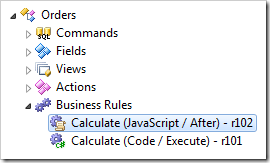Append the following:

 Property New Value Script ```[Total] = [Subtotal] + [Freight]; if ([Freight] || [Freight] == 0 || [Freight] == 3.95 || [Freight] == 19.95) { if ([Total] > 100) { [Freight] = 19.95; } else { [Freight] = 3.95; } }```

Press OK to save.

## Viewing the Results

On the toolbar, press Browse. Edit an order, and change the Freight to “0”. When you tab away from the field, the freight will be recalculated.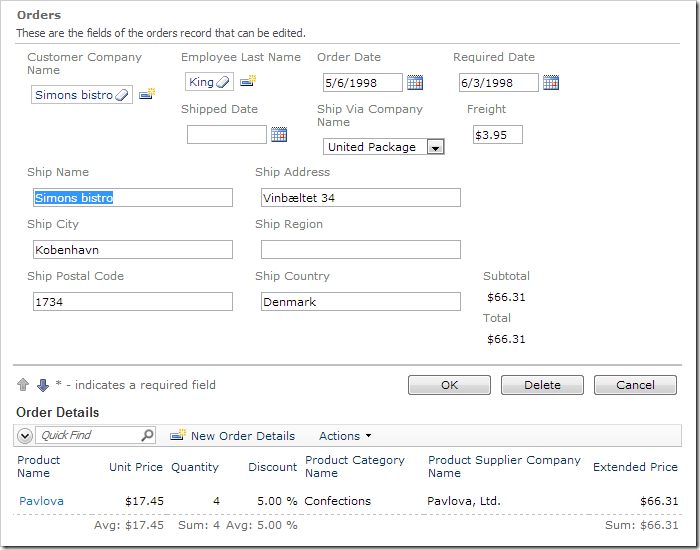If you change the order details so that the Subtotal is greater than \$100, you will see Freight be recalculated to \$19.95.Tuesday, October 30, 2012PrintSubscribe
Order Form Sample–Part 24

Currently, the new fields Total and Subtotal do not allow sorting or filtering. You can see this on the grid of Order Form – the Total column does not have a dropdown available, and the field is not displayed as an option in the advanced search bar.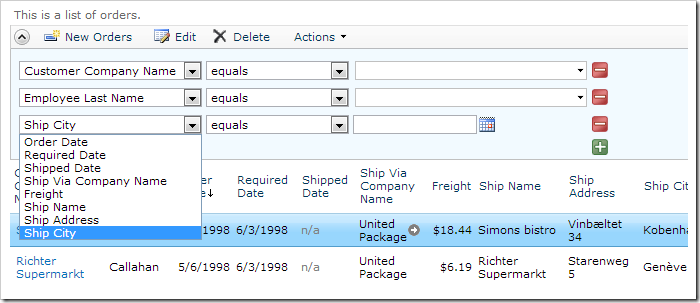Let’s enable sorting and filtering for these two fields. Switch back to the Project Explorer, and double-click on Orders / Fields / Subtotal* (Currency, read-only).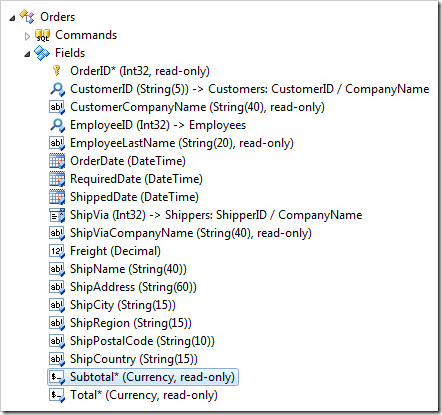Change the following settings:

 Property New Value Allow Query-by-Example true Allow Sorting true

Press OK to save the field. Now, double-click on Total field node.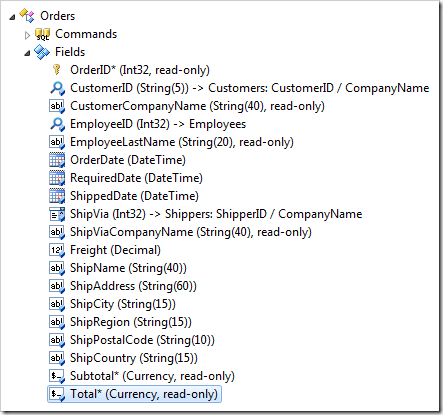Make the same changes:

 Property New Value Allow Query-by-Example true Allow Sorting true

Save the field, and regenerate the application. On the Order Form page, you can see that the Subtotal and Total fields are now available as options on the advanced search bar.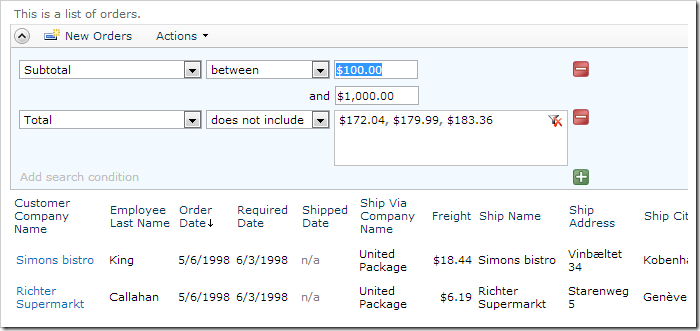Both columns also have sorting and filtering options available on the column header dropdown.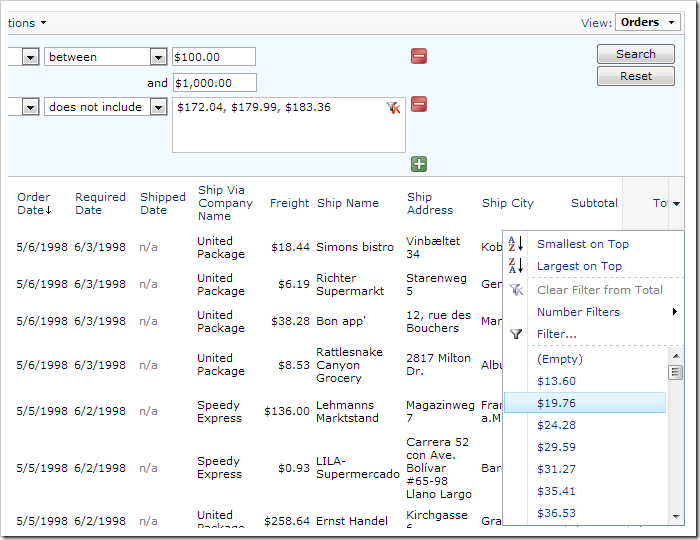Tuesday, October 30, 2012PrintSubscribe
Order Form Sample–Part 23

Let’s add a Total field to the Orders controller. This field will add Subtotal and Freight together to show the final cost of the whole order.

In the Project Explorer, right-click on Orders / Fields node. Press New Field.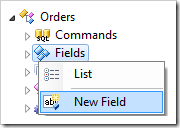Give this new field the following settings:

 Property Value Name Total Type Currency The value of this field is computed at run-time by SQL Expression true SQL Formula ```(select sum(UnitPrice*Quantity*(1-Discount)) from "Order Details" where "Order Details".OrderID = Orders.OrderID) + Orders.Freight ``` The value of this field is calculated by a business rule expression true Label Total Values of this field cannot be edited True Data Format String c Context Fields OrderDetails, Freight

Press OK to save this field.

## Binding the Field to Views

Drop Orders / Fields / Total* (Currency, read-only) field node onto Orders / Views / grid1 node.

Drop Total field node onto Orders / Views / editForm1 view node.

If you used an SQL Business Rule to handle Subtotal, double-click on Orders / Business Rules / Calculate (Sql / Execute) node.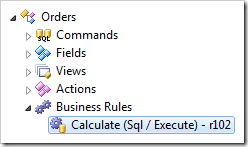Append the following highlighted line to the Script property:

 Property New Value Script ```select @Subtotal = sum(unitprice * quantity * (1 - discount)) from [Order Details] where OrderID = @OrderID set @Total = @Subtotal + @Freight```

If you are using C# or Visual Basic to handle the Subtotal field, right-click on Orders / Business Rules and press Edit Rule in Visual Studio.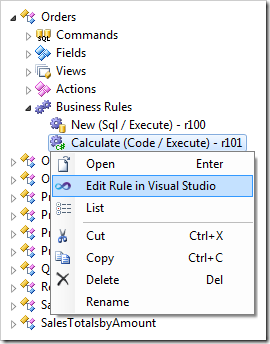Make the following changes to the code base:

C#:

```using System;
using MyCompany.Data;

namespace MyCompany.Rules
{
{

[Rule("r101")]
public void r101Implementation(
int? orderID,
string customerID,
string customerCompanyName,
int? employeeID,
string employeeLastName,
DateTime? orderDate,
DateTime? requiredDate,
DateTime? shippedDate,
int? shipVia,
string shipViaCompanyName,
decimal? freight,
string shipName,
string shipCity,
string shipRegion,
string shipPostalCode,
string shipCountry,
decimal? subtotal)
{
using (SqlText calc = new SqlText(@"select sum(unitprice *
quantity * (1 - discount)) from
[Order Details] where OrderID= @OrderID"))
{
object total = calc.ExecuteScalar();
if (DBNull.Value.Equals(total))
{
UpdateFieldValue("Subtotal", 0);
UpdateFieldValue("Total", 0);
}
else
{
UpdateFieldValue("Subtotal", Convert.ToDecimal(total));
UpdateFieldValue("Total", Convert.ToDecimal(total) + freight);
}
}
}
}
}```

Visual Basic:

```Imports MyCompany.Data
Imports System

Namespace MyCompany.Rules

<Rule("r101")> _
Public Sub r101Implementation( _
ByVal orderID As Nullable(Of Integer), _
ByVal customerID As String, _
ByVal customerCompanyName As String, _
ByVal employeeID As Nullable(Of Integer), _
ByVal employeeLastName As String, _
ByVal orderDate As Nullable(Of DateTime), _
ByVal requiredDate As Nullable(Of DateTime), _
ByVal shippedDate As Nullable(Of DateTime), _
ByVal shipVia As Nullable(Of Integer), _
ByVal shipViaCompanyName As String, _
ByVal freight As Nullable(Of Decimal), _
ByVal shipName As String, _
ByVal shipCity As String, _
ByVal shipRegion As String, _
ByVal shipPostalCode As String, _
ByVal shipCountry As String, _
ByVal subtotal As Nullable(Of Decimal))
Using calc As SqlText = New SqlText(
"select sum(unitprice * quantity * (1 - discount)) " +
"from [Order Details] where OrderID=@OrderID")
Dim total As Object = calc.ExecuteScalar()
If DBNull.Value.Equals(total) Then
UpdateFieldValue("Subtotal", 0)
UpdateFieldValue("Total", 0)
Else
UpdateFieldValue("Subtotal", Convert.ToDecimal(total))
UpdateFieldValue("Total", Convert.ToDecimal(total) + freight)
End If
End Using
End Sub
End Class
End Namespace
```

You can also implement a JavaScript business rule that will use the returned value of Subtotal and sum it with Freight to calculate the value of Total.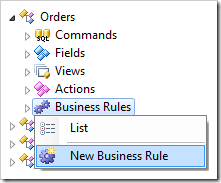Assign the following values:

 Property Value Type JavaScript Command Name Calculate Phase After Script `[Total] = [Subtotal] + [Freight];`

## Viewing the Results

On the toolbar, press Browse to regenerate the application. Navigate to the Order Form page, and select any order. You will see a Total field that sums up Subtotal and Freight.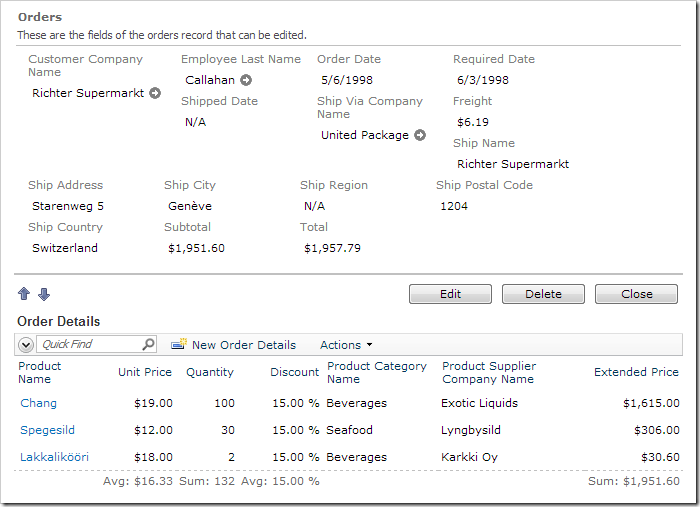When any of the order detail records are changed, the Subtotal and Total will be updated.